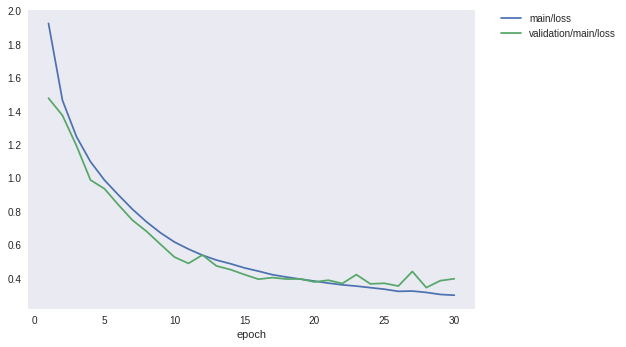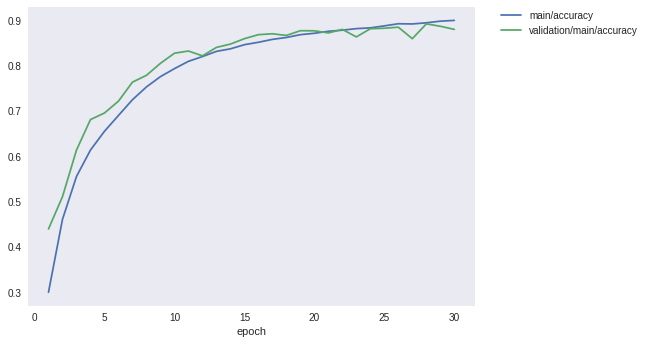How to write a custom dataset class¶

A typical strategy to improve generalization in deep neural networks is to increase the number of training examples which allows more parameters to be used in the model. Even if the number of parameters is kept unchanged, increasing the number of training examples often improves generalization performance.

Since it can be tedious and expensive to manually obtain and label additional training examples, a useful strategy is to consider methods for automatically increasing the size of a training set. Fortunately, for image datasets, there are several augmentation methods that have been found to work well in practice. They include:

• Randomly cropping several slightly smaller images from the original training image.
• Horizontally flipping the image.
• Randomly rotating the image.
• Applying various distortions and or noise to the images, etc.

In this example, we will write a custom dataset class that performs the first two of these augmentation methods on the CIFAR10 dataset. We will then train our previous deep CNN and check that the generalization performance on the test set has in fact improved.

We will create this dataset augmentation class as a subclass of DatasetMixin, which has the following API:

• __len__ method to return the size of data in dataset.
• get_example method to return data or a tuple of data and label, which are passed by i argument variable.

Other necessary features for a dataset can be prepared by inheriting chainer.dataset.DasetMixin class.

:

# Install Chainer and CuPy!

!curl https://colab.chainer.org/install | sh -

Reading package lists... Done
Building dependency tree
0 upgraded, 0 newly installed, 0 to remove and 1 not upgraded.
Requirement already satisfied: six>=1.9.0 in /usr/local/lib/python3.6/dist-packages (from cupy-cuda80==4.0.0b3)
Requirement already satisfied: fastrlock>=0.3 in /usr/local/lib/python3.6/dist-packages (from cupy-cuda80==4.0.0b3)
Requirement already satisfied: numpy>=1.9.0 in /usr/local/lib/python3.6/dist-packages (from cupy-cuda80==4.0.0b3)
Requirement already satisfied: chainer==4.0.0b3 in /usr/local/lib/python3.6/dist-packages
Requirement already satisfied: protobuf>=3.0.0 in /usr/local/lib/python3.6/dist-packages (from chainer==4.0.0b3)
Requirement already satisfied: filelock in /usr/local/lib/python3.6/dist-packages (from chainer==4.0.0b3)
Requirement already satisfied: six>=1.9.0 in /usr/local/lib/python3.6/dist-packages (from chainer==4.0.0b3)
Requirement already satisfied: numpy>=1.9.0 in /usr/local/lib/python3.6/dist-packages (from chainer==4.0.0b3)
Requirement already satisfied: setuptools in /usr/lib/python3/dist-packages (from protobuf>=3.0.0->chainer==4.0.0b3)


1. Write the dataset augmentation class for CIFAR10¶

:

import numpy as np
from chainer import dataset
from chainer.datasets import cifar

gpu_id = 0  # Set to -1 if you don't have a GPU

class CIFAR10Augmented(dataset.DatasetMixin):

def __init__(self, train=True):
train_data, test_data = cifar.get_cifar10()
if train:
self.data = train_data
else:
self.data = test_data
self.train = train
self.random_crop = 4

def __len__(self):
return len(self.data)

def get_example(self, i):
x, t = self.data[i]
if self.train:
x = x.transpose(1, 2, 0)
h, w, _ = x.shape
x_offset = np.random.randint(self.random_crop)
y_offset = np.random.randint(self.random_crop)
x = x[y_offset:y_offset + h - self.random_crop,
x_offset:x_offset + w - self.random_crop]
if np.random.rand() > 0.5:
x = np.fliplr(x)
x = x.transpose(2, 0, 1)
return x, t

/usr/local/lib/python3.6/dist-packages/cupy/core/fusion.py:659: FutureWarning: cupy.core.fusion is experimental. The interface can change in the future.
util.experimental('cupy.core.fusion')


This class performs the following types of data augmentation on the CIFAR10 example images:

• Randomly crop a 28X28 area form the 32X32 whole image data.
• Randomly perform a horizontal flip with 0.5 probability.

2. Train on the CIFAR10 dataset using our dataset augmentation class¶

Let’s now train the same deep CNN from the previous example. The only difference is that we will now use our dataset augmentation class. Since we reuse the same model with the same number of parameters, we can observe how much the augmentation improves the test set generalization performance.

:

import chainer
import chainer.functions as F
from chainer.datasets import cifar
from chainer import iterators
from chainer import optimizers
from chainer import training
from chainer.training import extensions

class ConvBlock(chainer.Chain):

def __init__(self, n_ch, pool_drop=False):
w = chainer.initializers.HeNormal()
super(ConvBlock, self).__init__()
with self.init_scope():
self.conv = L.Convolution2D(None, n_ch, 3, 1, 1,
nobias=True, initialW=w)
self.bn = L.BatchNormalization(n_ch)

self.pool_drop = pool_drop

def __call__(self, x):
h = F.relu(self.bn(self.conv(x)))
if self.pool_drop:
h = F.max_pooling_2d(h, 2, 2)
h = F.dropout(h, ratio=0.25)
return h

class LinearBlock(chainer.Chain):

def __init__(self):
w = chainer.initializers.HeNormal()
super(LinearBlock, self).__init__()
with self.init_scope():
self.fc = L.Linear(None, 1024, initialW=w)

def __call__(self, x):
return F.dropout(F.relu(self.fc(x)), ratio=0.5)

class DeepCNN(chainer.ChainList):

def __init__(self, n_output):
super(DeepCNN, self).__init__(
ConvBlock(64),
ConvBlock(64, True),
ConvBlock(128),
ConvBlock(128, True),
ConvBlock(256),
ConvBlock(256, True),
LinearBlock(),
LinearBlock(),
L.Linear(None, n_output)
)

def __call__(self, x):
for f in self.children():
x = f(x)
return x

def train(model_object, batchsize=64, gpu_id=gpu_id, max_epoch=20):

# 1. Dataset
train, test = CIFAR10Augmented(), CIFAR10Augmented(False)

# 2. Iterator
train_iter = iterators.SerialIterator(train, batchsize)
test_iter = iterators.SerialIterator(test, batchsize, False, False)

# 3. Model
model = L.Classifier(model_object)
if gpu_id >= 0:
model.to_gpu(gpu_id)

# 4. Optimizer
optimizer.setup(model)

# 5. Updater
updater = training.StandardUpdater(train_iter, optimizer, device=gpu_id)

# 6. Trainer
trainer = training.Trainer(updater, (max_epoch, 'epoch'), out='{}_cifar10augmented_result'.format(model_object.__class__.__name__))

# 7. Evaluator

class TestModeEvaluator(extensions.Evaluator):

def evaluate(self):
model = self.get_target('main')
ret = super(TestModeEvaluator, self).evaluate()
return ret

trainer.extend(extensions.LogReport())
trainer.extend(TestModeEvaluator(test_iter, model, device=gpu_id))
trainer.extend(extensions.PrintReport(['epoch', 'main/loss', 'main/accuracy', 'validation/main/loss', 'validation/main/accuracy', 'elapsed_time']))
trainer.extend(extensions.PlotReport(['main/loss', 'validation/main/loss'], x_key='epoch', file_name='loss.png'))
trainer.extend(extensions.PlotReport(['main/accuracy', 'validation/main/accuracy'], x_key='epoch', file_name='accuracy.png'))
trainer.run()
del trainer

return model

model = train(DeepCNN(10), gpu_id=gpu_id, max_epoch=30)

epoch       main/loss   main/accuracy  validation/main/loss  validation/main/accuracy  elapsed_time
1           1.92407     0.299832       1.47721               0.43959                   85.7994
2           1.46391     0.460767       1.37372               0.510947                  170.782
3           1.24698     0.555558       1.19284               0.613953                  255.058
4           1.09747     0.613997       0.987924              0.68133                   339.642
5           0.987182    0.655391       0.935555              0.69586                   424.341
6           0.898338    0.690401       0.839072              0.722034                  509.142
7           0.81304     0.725192       0.747639              0.764033                  593.771
8           0.738109    0.753441       0.681274              0.77906                   678.347
9           0.672411    0.776235       0.60375               0.805633                  762.626
10          0.617099    0.793834       0.527143              0.827926                  846.95
11          0.575074    0.810059       0.489332              0.832803                  931.496
12          0.538337    0.820563       0.539499              0.822154                  1016.05
13          0.509321    0.832021       0.474118              0.840764                  1101.08
14          0.487421    0.837628       0.452331              0.848129                  1185.79
15          0.462403    0.846771       0.422598              0.860072                  1270.2
16          0.442934    0.852153       0.394928              0.868929                  1354.51
17          0.421746    0.858516       0.404235              0.87092                   1438.79
18          0.407674    0.862756       0.395771              0.867237                  1523.3
19          0.39542     0.868938       0.396354              0.877687                  1607.85
20          0.383477    0.871999       0.37822               0.877488                  1692.17
21          0.371322    0.876399       0.388828              0.87281                   1777.04
22          0.36101     0.878861       0.369287              0.880573                  1861.17
23          0.353587    0.882282       0.422225              0.863953                  1936.13
24          0.344247    0.883963       0.366904              0.882066                  1977.98
25          0.335103    0.888387       0.370403              0.883161                  2019.74
26          0.322226    0.893086       0.353938              0.88545                   2061.59
27          0.323977    0.892626       0.44101               0.860072                  2103.4
28          0.315712    0.895146       0.344539              0.892815                  2145.16
29          0.303662    0.898777       0.385994              0.88754                   2187.08
30          0.29911     0.900448       0.397462              0.880573                  2228.82


In the case without the previous data augmentation, it was found that the precision which was capped at about 87% can be improved to 89% or more by applying augmentation to the learning data. It is an improvement of over 2%.

Finally, let’s take a look at the loss and precision graph.

:

from IPython.display import Image
Image(filename='DeepCNN_cifar10augmented_result/loss.png')

::

Image(filename='DeepCNN_cifar10augmented_result/accuracy.png')

: